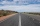# Broken tree

The tree was 35 meters high. The tree broke at a height of 10 m above the ground. Top but does not fall off it refuted on the ground. How far from the base of the tree lay its peak?

Result

x =  22.913 m

#### Solution:Leave us a comment of example and its solution (i.e. if it is still somewhat unclear...):

Showing 0 comments:Be the first to comment!#### To solve this example are needed these knowledge from mathematics:

Pythagorean theorem is the base for the right triangle calculator.

## Next similar examples:

1. Broken treeThe tree is broken at 4 meters above the ground and the top of the tree touches the ground at a distance of 5 from the trunk. Calculate the original height of the tree.
2. WindbreakA tree at a height of 3 meters broke in the windbreak. Its peak fell 4.5 m from the tree. How tall was the tree?
3. Double ladderThe double ladder is 8.5m long. It is built so that its lower ends are 3.5 meters apart. How high does the upper end of the ladder reach?
4. Four ropesTV transmitter is anchored at a height of 44 meters by four ropes. Each rope is attached at a distance of 55 meters from the heel of the TV transmitter. Calculate how many meters of rope were used in the construction of the transmitter. At each attachment.
5. LadderThe ladder has a length 3.5 meters. He is leaning against the wall so that his bottom end is 2 meters away from the wall. Determine the height of the ladder.
6. Double ladderThe double ladder shoulders should be 3 meters long. What height will the upper top of the ladder reach if the lower ends are 1.8 meters apart?
7. Horizontal distanceThe road has a gradient of 8%. How many meters will the road rise on a horizontal distance of 400m?
8. StairwayStairway has 20 steps. Each step has a length of 22 cm and a height of 15 cm. Calculate the length of the handrail of staircases if on the top and bottom exceeds 10 cm.
9. Cone 15The radius of the base of a right circular cone is 14 inches and it's height 18 inches. What is the slant height?
10. Chord circleThe circle to the (S, r = 8 cm) are different points A, B connected segment /AB/ = 12 cm. AB mark the middle of S'. Calculate |SS'|. Make the sketch.
11. Rectangular triangle PQRIn the rectangular triangle PQR, the PQ leg is divided by the X point into two segments of which longer is 25cm long. The second leg PR has a length 16 cm. The length of the RX is 20 cm. Calculate the length p of side RQ. The result is round to 2 decimal
12. Common chordTwo circles with radius 17 cm and 20 cm are intersect at two points. Its common chord is long 27 cm. What is the distance of the centers of these circles?
13. Trio 2Decide whether trio of numbers is the side of a right triangle: 26,24,10.
14. SatinSanusha buys a piece of satin 2.4 m wide. The diagonal length of the fabric is 4m. What is the length of the piece of satin?
15. EnrollmentThe enrollment at a local college increased 4% over last year's enrollment of 8548. Find the increase in enrollment (x1) and the current enrollment (x2).
16. Waiting roomIn the waiting room are people and flies. Together they have 15 heads and 50 legs (fly has 6 legs). How many people and flies are in the waiting room?
17. Divisible by 5How many three-digit odd numbers divisible by 5, which are in place ten's number 3?